# Quantum harmonic oscillator tunneling puzzle

In summary, the animation presents a problem with a quantum harmonic oscillator. The problem is that there is a nonzero probability of finding the oscillating point in a classically forbidden region. The tunneling probability decreases as the quantum number n increases.

My problem is described in the animation that I posted on Youtube:

For the sake of convenience I am copying here the text that follows the animation:

I have made this animation in order to present my little puzzle with the quantum harmonic oscillator. Think about a classical oscillator, a swing, a weight on a spring, a pendulum in a clock ... It has a certain characteristic spring constant, and a mass. For simplicity let us choose units so that these constants are numerically equal to 1. Let us also assume that the time scale is choosen so that the period is equal 2 pi. Given energy E, the classical oscillator will vibrate with an amplitude A. Its deviation x(t) from the equilibrium position x=0 is given by the formula

x(t) = A cos t

The relation between energy E and amplitude A is simple: square of A is 2E. For a classical oscillator the energy E can be any positive number.

For a quantum oscillator, assuming units in which the Planck constant hbar = 1, the energy can not be arbitrary, it is quantized: 2E(n) = 2n+1. To each energy level there corresponds a "quantum eigenstate", the "wave function". Using Mathematica notation it is given by the formula

psi[n_,x_] = 1/Sqrt[Sqrt[Pi] 2^n n!] Exp[-x^2/2] HermiteH[n, x]

This wave function (notice that it is real-valued) is normalized so that its square gives the probability density of finding the oscillating point (with energy E(n)) at the point x. Classically we will never find x beyond the turning ponts xmin = -A and xmax = A. But for our quantum oscillator there is always a nonzero probability of finding the point in a classically forbidden region. We say that that there is a non-zero tunneling probability.

Now the puzzle:

Here is a relevant sentence from one of the popular textbooks:

"The probability of being found in classically forbidden regions decreases quickly with increasing n, and vanishes entirely as n approaches inﬁnity, as we would expect from the correspondence principle."

"Quanta, Matter and Change. A molecular approach to physical chemistry", P.W. Atkins, J. de
Paula and R.S. Friedman, Oxford University Press, Oxford/New York, 2009, p. 66

So I used Wolfram's Mathematica to calculate these tunnelling probabilities for n = 0,...,512. The calculation have been done symbolically in order to avoid as much as possible numerical errors.
The animation shows the sequence of plots of probability densities, the classical limits, and also, in a sub-window, the tunelling probability for each n. There is also an U shaped curve representing the classical probability density of finding the swing at a given position given only its energy, but not knowing its phase. In the animation each graph is scaled so that the classical turning points are always at x=-1 and x=1. The vertical axis is also scaled so that the total probability (the area under the probability densities) is always 1.

It can be seen that indeed, the tunneling probability, at first, decreases rather quicly, but then its decrease rate is essentially slowing down with the increase of the quantum number n. This is puzzling. It is a surprise. From my correspondence with one of the authors of the quoted monograph I know that it is also a surprise for him. For instance, I could not find any estimate of what n is needed to have the tunneling probability smaller than, say, 1/1000000? Is it known?

•wabbit
Hi Arkadiusz, and welcome back to PF. I don't there's a 'hidden' explanation to this issue. It's just (simple) mathematics and a particular behavior of a 1-variable function which is found starting with the known and accepted principles of quantum mechanics, the same principles that led us (through QFT) to the most successful (perturbative & not mathematically rigorous) theory we still have nowadays. QED and its famous g-2 accuracy.

As for your particular finding here, it's for me just another proof that making unsubstantiated claims in textbooks is a pretty intelectually dangerous activity.

What's missing is perspective. Consider diatomic oxygen, it has a vibrational zero point energy of $790cm^{-1}$ which is roughly $1\times 10^{-23}J$. Let's call a classical energy one joule. Then to get to the classical regime the quantum number must be on the order of $n \approx 10^{23}$! A long way to go from 500.

I was able to calculate the "tunneling probabilty" analytically in the limit of large ##n##. The result I got was ##c n^{-1/3}## where ##c=\Gamma[1/3]/(2^{5/3}3^{2/3}\pi)=0.129##. This appears to match your curve.

Thanks, Avodyne. This was also my guess. but only guess. A week ago I posted the numerical fit based on the first 100 points on my blog: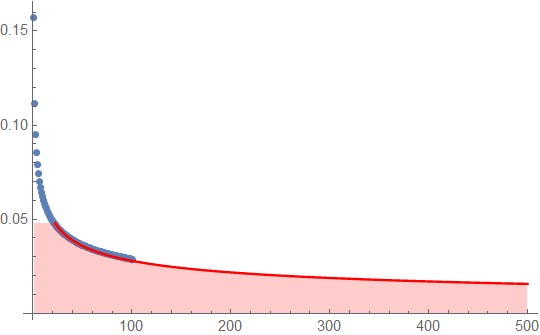My fit was a/n^k, a = 0.1465, k = 0.359871. Your formula matches the asymptotic part better.

It would be useful (for instance for using in textbooks) to see the details of the arguments used in your theoretical derivation. Would you like to post it?

Haborix, if we accept the asymptotic formula by Avodyne, then to get the quantum tunneling probability less than 1/1000000 we need n of the order of $10^{15}.$
Indeed A long way to go from 500.

dextercioby: Looking at the first 30-40 probability density plots one gets indeed the impression of a quick descend of the nonclassical tail areas. Textbooks usually stop at that, as for instance in Thaller, Visual Quantum Mechanics, Springer (2000), p. 168: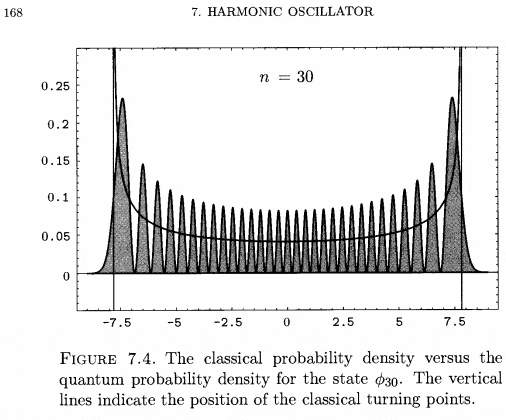Further calculations need more number crunching, so that the wrong impression of a fast descend of tunneling probabilities is being formed.

To do the calculation, I used the asymptotic formula for the (unnormalized) quantum wave function found here:
http://en.wikipedia.org/wiki/Hermite_polynomials#Asymptotic_expansion
(3rd equation from the end of this section). Normalize the wave function correctly, square it, change integration variables from ##x## to ##\phi##, and integrate over ##\phi>0##. The exponent ##2\phi-\sinh(2\phi)## can be replaced by the first term in its Taylor expansion, ##-4\phi^3/3##, to get the leading behavior of the integral.

I'm also surprised by the slow fall off with ##n##, because the wave function falls exponentially fast in the forbidden region. However, it's also starting from a height that goes to infinity in the classical limit. Apparently these two effects offset.

Avodyne, I am trying to understand your derivation. I checked Wiki, then went to the cited monograph by Szego. Szego is somewhat more precise than Wikipedia: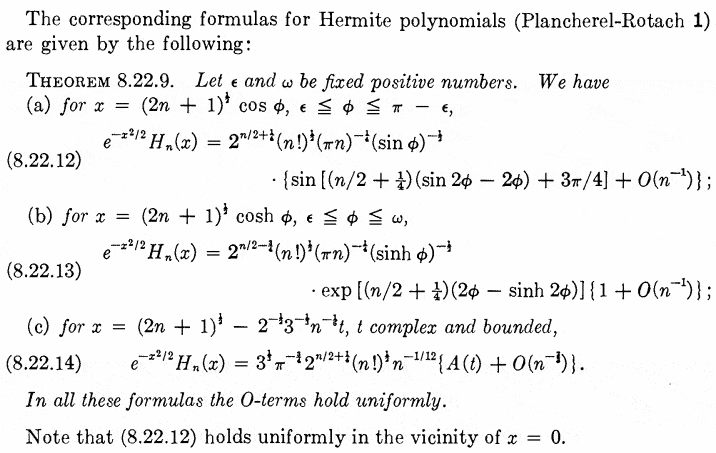Do you mean you were using (8.22.13) , which is supposed to be valid only for $x>=(2n+1)^{1/2}$, and applied it to the whole real axis?

For completeness: here is the comparison of the calculated probabilities with Avodyne's asymptotic formula (in red), for $100\le n\le 512$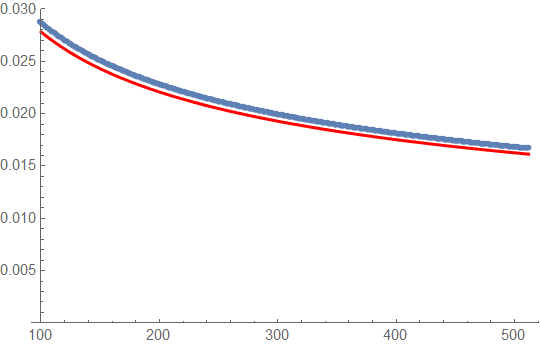Do you mean you were using (8.22.13) , which is supposed to be valid only for $x>=(2n+1)^{1/2}$, and applied it to the whole real axis?
No, I used it only for ##x\ge(2n+1)^{1/2}##. This is the classically forbidden region. So I computed
$$\int_{\sqrt{2n+1}}^\infty|\psi(x)|^2 dx$$ using (8.22.13) to approximate ##\psi(x)##.

Update: in order to look at it from a different angle I did the animation for the Wigner quasiprobability distribution

Details are in the description on Youtube. Here the meaning of the clasically forbidden tails is less evident.

Avodyne, if you expanded only the tails, how did you normalize? Afterwards? Did you normalize $n^{-1/3}$?

The eigenfunction is ##\psi_n(x)=N H_n(x)e^{-x^2/2}## with ##N=\pi^{-1/4}(2^n n!)^{-1/2}##. Eq.(8.22.13) gives an approximation to ##H_n(x)e^{-x^2/2}## that is valid for ##x>\sqrt{2n+1}##. I multiplied this by ##N## to get an approximation to ##\psi_n(x)## valid for ##x>\sqrt{2n+1}##.

OK. Thanks. All clear now. Evidently you also used $dx/d\phi = \sqrt{2n+1}\sinh\phi$

Last edited:
Avodyne: I am trying to reconstruct your argument. Yet I am getting a different coefficient, approximately twice your value. Perhaps I am making some mistake. Would you like to look at my calculations in the pdf document here?

Update: I double checked my calculations. Could not find a mistake. Therefore my hypothesis is as follows: while the shape of the asymptotic may indeed be like $n^{-1/3}$, the constant factor can only be found through a fit to the exact data. One must not expect a proper probability normalization constant from the asymptotic behavior in a particular region. Shall we agree with such a proposal?

Last edited:
I think you lost a factor of 2 between your (16) and (17). I claim that, in the large ##n## limit, your ##d_n##, (13), is just ##1/(2\pi)##. But the ratio between (17) and (16), which should be ##d_n##, is ##1/\pi## (again in the large ##n## limit).

Uploaded a new version with several misprints corrected, including missing 2 in (13). Checking again.

Update: our results indeed differ by the factor $2$. Are you sure you multiplied by 2 in order to take into account both tails, left and right?

Last edited:
OK, I've realized several things.

First, I was not multiplying by 2 to get both forbidden regions.

Second, after I do this, my result is numerically too large by about a factor of 2. I checked this by comparing it to the actual probability for ##n=100##.

Third, that asymptotic formula we're both using is actually only valid for values of ##\phi## that are pretty big, ##\phi>0.25## for ##n=100##. For smaller values of ##\phi##, where the bulk of the tunnelling probability comes from, it's actually a terrible approximation. It's only good for getting the extreme tail of the wave function.

Fourth, the "transition" formula, eq.(8.22.14), works well in the tunnelling region near the boundary of the allowed region, so this is the one to use. I'm not sure what Szego's ##A(t)## is, so I'm using the wikipedia version of the formula, which involves a standard Airy function. Plotting this approximation and the exact wave function for ##n=100##, the curves lie right on top of each other in the forbidden region.

Finally, using the "transition" formula, I get the probability that ##|x|>\sqrt{2n+1}## is ##cn^{-1/3}##, where ##c=2/(3^{2/3}\Gamma[1/3]^2)=0.133975##. So the same ##n## dependence as before, but now with a coefficient that matches the numerical results well.

EDIT: here is the approximate function I'm now using for the normalized energy eigenfunction:
$$\psi_n(x)\simeq 2^{1/4}n^{-1/12}\mathop{\rm Ai}\bigl(2^{1/2}n^{1/6}(x-\sqrt{2n+1})\bigr)$$where the Airy function ##{\rm Ai}(x)## is defined here: http://en.wikipedia.org/wiki/Airy_function
In Mathematica, it is AiryAi[x].

Last edited:
Thanks a lot for your thoughts and for your work! I will play now with Airy in order to reproduce your results.

Here's a plot (via Mathematica) of the exact ##\psi_{100}(x)## (blue) and the approximation (red). The x-axis is ##x/\sqrt{201}##, so the forbidden region is to the right of 1.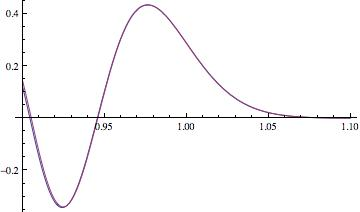Looks surprisingly good indeed. Hopefully the integrals also agree. Will try to reproduce your results.

Szego's A and Airy Ai:
$$A(x)=3^{-1/3}\pi\mbox{Ai}(-3^{-1/3}x)$$
Source: Olver et al, NIST Handbook of Mathematical Functions, (2010), p. 194

Avodyne, I reproduced your results, updated the pdf file (please, verify). The formula is really amazing. Here is the plot of the calculated tunneling probabilities from the exact formula (blue) and the curve obtained using Airy functions (red). I plotted both starting from n=5 !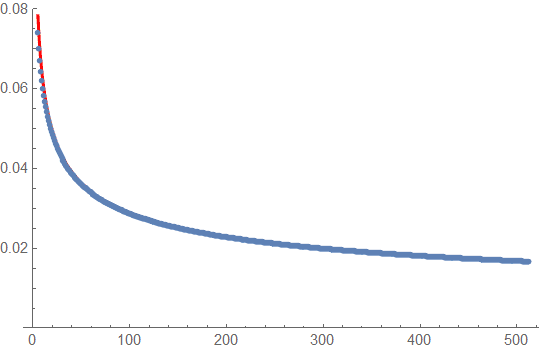I agree with your calculations. But I would say that (8.22.13) was never relevant. Here is a plot, for n=100, of the exact (blue), Airy approx (8.22.14) (red), and (8.22.13) (yellow) of the probability density (normalized wave-function squared) in the forbidden region; x-axis is ##x/\sqrt{201}##: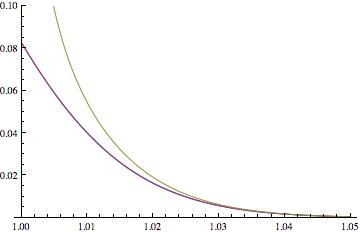So we see that (8.22.13) is quite bad, and when integrated will give a probability that is much too large. (8.22.13) only becomes good out on the tail: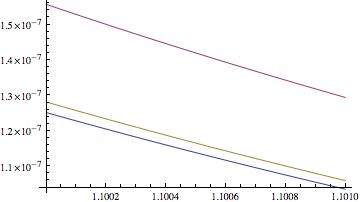But out here, the probability density is so small that we don't care that the yellow line is a much better approx than the red line.

I agree. Yet there is till one unsolved problem. Wikipedia (following Szego) tells us that the error estimate in the Airy function approximation is valid for "t bounded". But we are using the formula for the unbounded region, extending to infinity. The results came out to be good, but a real mathematical justification is still somewhat problematic.

Avodyne,
Since I did not see this problem discussed in depth anywhere, and since it seems to be interesting as it deals with the properties of one of the most fundamental models in quantum mechanics, perhaps you would like to write a short paper on this subject? These results should be available for authors of future textbooks.

P.S. I calculated with Mathematica the "exact" tunelling probability for n=612. The result is 0.00787792, while the Airy formula predicts 0.01578. So, we have a discrepancy. But for $n>512$ I do not believe the "exact" calculation any more. Numbers involved are probably too big, more refined methods and higher precision is probably necessary.

Last edited:
I'm sure a real mathematical justification is possible. Don't integrate to infinity, but stop at large but finite ##t## (in Szego's notation). Then bound the value of the integrated tail that is not included. I'm sure it can be shown to go to zero faster than ##n^{-1/3}##, and so can be dropped.

I agree that Mathematica is not trustworthy for ##n## too large without special methods, too many cancellations in the Hermite polynomial.

Not interested in writing a paper, but feel free. If you do, you can put my name in the acknowledgments for discussions (my real name, which I'll send you if you write the paper!).

Thanks. Increasing the precision Mathematica was able to give a reasonable number also for n=612: 0.01575584. Perhaps I will now look for some help from expert mathematicians to be check if in this particular case the global asymptotic formula can be justified.

Update: computed 100 additional points. Plotted the asymptotic formula vs numerical "exact data". The agreement seems to be too good to be accidental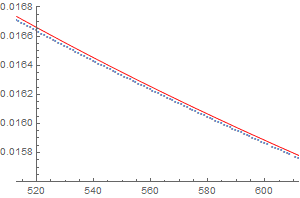and yet a solid mathematical foundation is still missing.

Avodyne: Please check the new file .
The paper is at the final stages. there is still an "anonymous helper" in the acknowledgments.

Last edited by a moderator:
So would I be right in thinking that this is no longer a puzzle but just a slight surprise?Actually I found the result a little surprising myself. I think it was because the probability density is a function of both x and n. I naturally expected an exponential dependence on x and I guess this spilled over to expecting a steep dependence on n as well. But as soon as I realized this, I started wondering whether there was any intuitive reason to expect it to fall off with n at all - it would not, AFAIK, go against any fundamental principle if the probability of finding a system outside of its classical range asymptotically approached some constant.

The old problem of large quantum numbers and the correspondence principle is still being discussed. Cabrera and Kiwi, Large quantum-number states and the correspondence principle, Phys. Rev. A 36, 2995(R) September 1987 show how it can be violated for the harmonic oscillator.